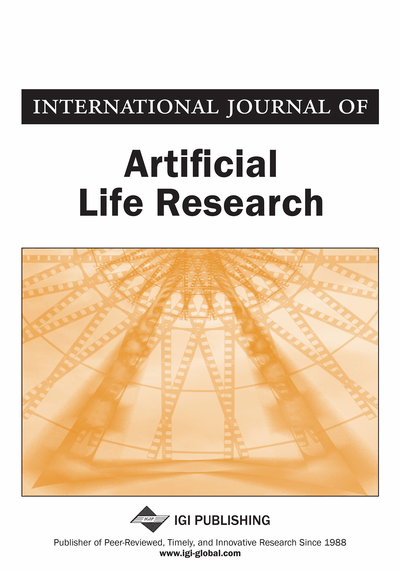# Super-Efficiency DEA Approach for Optimizing Multiple Quality Characteristics in Parameter Design

Abbas Al-Refaie (University of Jordan, Jordan)
DOI: 10.4018/jalr.2010040105
Available
\$33.75
List Price: \$37.50
10% Discount:-\$3.75
TOTAL SAVINGS: \$3.75

## Abstract

This paper proposes an efficient approach for optimizing the multiple quality characteristics (QCHs) in manufacturing applications on the Taguchi method using the super efficiency technique in data envelopment analysis (DEA). Each experiment in Taguchi’s orthogonal array (OA) is treated as a decision making unit (DMU) with multiple QCHs set as inputs or outputs. DMU’s efficiency is measured then adopted as a performance measure to identify the combination of optimal factor levels. Three real case studies were employed for illustration in which the proposed approach provided the largest total anticipated improvements in multiple QCHs among other techniques such as principal component analysis (PCA) and DEA based ranking (DEAR) approach. Analysis of variance is finally employed to decide significant factor effects and to predict performance.
Article Preview
Top

## Introduction

The overall goal of robust design is to find settings of the controllable factors so that the QCH is least sensitive to variations in the noise variables, while still yielding an acceptable mean level of the QCH. A particularly cost-effective approach is the robust design introduced by Taguchi (1991), which aims for finding the optimum settings of control factors to make the product or process insensitive to noise factors. Typically, the QCH is divided into three main types; the smaller-the-better (STB), the nominal-the-best (NTB), and the larger-the-better (LTB) type QCHs. In the Taguchi method, an orthogonal array (OA) is utilized to reduce the number of experiments under permissive reliability. Signal-to-noise (S/N) ratio is then adopted to optimize performance. Although this method has been widely applied, it is usually efficient for only optimizing a single QCH. Recently, optimization of multiple QCHs in the Taguchi method was faced by several studies (Tong et al., 1997; Lin & Lin, 2002; Jean & Wang, 2006; Al-Refaie et al., 2008; Al-Refaie et al., 2009).

Data envelopment analysis (DEA) developed by Charnes et al. (1978) is a fractional mathematical programming technique widely used for measuring the performance of homogeneous decision making units (DMUs) with multiple inputs and multiple outputs at organizational level; such as cities, hospitals, and universities The most popular DEA technique is the CCR model developed by Charnes, Cooper, and Rhodes (1978). Assuming there are n DMUs each with m inputs and s outputs to be evaluated, then the CCR model measures the relative efficiency of each DMU once by comparing it to a group of the other DMUs that have the same set of inputs and outputs. Hence, there are n optimizations needed. The relative efficiency, Eo, of DMUo with inputs of Xio (i = 1, ..., m) and outputs of Yro (r = 1, ..., s) is evaluated by CCR model as (Charnes et al., 1994)

(1) where Yrj is the rth output for DMU j, Xij is the ith input for DMU j, Ur is the weight given to the rth output, Vi is the weight assigned to the ith input, and θ is a scalar. The objective function is to maximize the efficiency of DMU being evaluated, DMUo, subject to the relative efficiency, DMUj (j = 1,…, n) is less or equal to one with positive virtual weights. The CCR model identifies DMUo efficient as if Eo equals one. Otherwise, DMUo is identified as CCR-inefficient. Obviously, the CCR model is nonlinear, which can be converted into a linear programming problem by considering the “input-oriented” CCR model expressed as

(2)

## Complete Article List

Search this Journal:
Reset
Open Access Articles: Forthcoming
Volume 8: 2 Issues (2018)
Volume 7: 2 Issues (2017)
Volume 6: 2 Issues (2016)
Volume 5: 1 Issue (2015)
Volume 4: 1 Issue (2014)
Volume 3: 4 Issues (2012)
Volume 2: 4 Issues (2011)
Volume 1: 4 Issues (2010)
View Complete Journal Contents Listing